# Fun numpy things

Diff two images

diff_image = abs(prediction_mask - true_mask)

Count pixels that exactly match between two images

diff_image = abs(prediction_mask - true_mask)
matches = 1.0 * (diff_image == 0)
matches_count = np.sum(matches)

Count prediction pixels that do not match truth-mask pixels

diff_image = abs(prediction_mask - true_mask)
unpaired_pred_msk  = 1.0 * (prediction_mask>0) * (diff_img>0)
un_matched_pred_count = np.sum(unpaired_pred_msk)


# So what is numpy Matrix Multiplication?

What is the product of two matrices?

What is the dot product of two matrices?

What is the multiplication of two matrices?

I studied linear algebra in collage in 1984. I wrote my first computer program in Fortran in 1982 using punch cards. I wrote my first matrix multiplication program on an Texas Instruments Calculator in 1985. I started working with numpy matrices in 2019, which is 36 computer-age-years later.

At my first attempt at numpy matrix multiplication I did not get the expected results. As I dug into the documenation, I was very confused because the use of the words “multiply” and “matrix” have different meanings between my text book from late 20th century and early 21st century numpy.

Since I have not studied mathematics formally since completing my masters in the 1990s, I will not claim the linear algebra definitions have not changed, I will only refer to the “text book” I used in the 1980s.

Here is what I learned: numpy matrix multiplication is not mathematical matrix multiplication. But the numpy dot product is what I learned as matrix multiplication back in 1984, sort of….

## Text book definition

Here is my text book definition of the “product” of two matrices.

Notice the introduction to the concept at the top of the page, I quote:

Perhaps the most natural definition of matrix multiplication would seem to be: “multiply corresponding entries together.” Surprisingly, however, this definition would not be very useful for most problems.

P 26, Elementary Linear Algebra, Fourth Edition, by Howard Anton

The author explicitly says multiplying corresponding elements is not the definition of matrix multiplication, but this is precisely the numpy definition of matrix multiplication.

However, numpy does provide a mathematically correct method to compute the product of two matrices, referred to as the ‘dot’ product. To confuse matters more, in my text book, dot product refers only to vectors (1D matrices). In numpy, it refers to N-D matrices.

## Definition of numpy Matrix Multiplication

For reference:

https://numpy.org/doc/stable/reference/generated/numpy.multiply.html

The numpy.multiply() function simply multiplies corresponding elements. This requires that arrays have the same dimensions. If you attempt to multiply two matrices of different dimensions, numpy will either ‘broadcast’ the array to create a matching size, or throw an error if broadcasting will not work. For example:

Given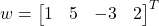andWhat is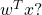numpy array math is not standard mathmatics. As a python numpy developer you have to be very aware of the nuances of lists of numbers, lists of lists, numpy vectors and numpy arrays as the results you get on common operations will differ.

In this example I will only discuss the case of mumpy matrices (array with two dimensions). So, in python, using standard multiplication (* == np.multiply()):

>>> import numpy as np
>>> w = np.array([[1,5,-3,2] ]).transpose()    # defined as a numpy 2-D array of 1 x 4, if we used vectors,
>>> x = np.array([ [8,2,4,7] ]).transpose()    #  the transpose would not work
>>> wt = w.transpose()
>>> wtx = wt * x
>>> wtx
array([[  8,  40, -24,  16],
[  2,  10,  -6,   4],
[  4,  20, -12,   8],
[  7,  35, -21,  14]])

Notice we get a 4 x 4 matrix as a result. Not at all what we expect from the text book definition. The text book definition of multiplying a 1 x 4 vector with a 4 x 1 vector is a 1 dimensional entity, a scalar (in the example, the result should be the value of 20).

What happened was numpy first broad cast the arrays to match dimensions:becomes:and,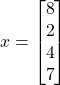becomes:So simply multiplying the corresponding elements we get a 4 x 4 matrix: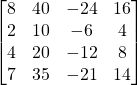## Getting the Mathematically Correct Answer

To get the text book correct result, just use the numpy dot() method:

>>> wtx = wt.dot(x)
>>> wtx
array([])

But be aware, the correct format of the answer should be the scaler value 20, which numpy displays a 1 x 1 matrix.

# Mathematical Definition of a Dot Product

The dot product of two vectorsandis a scaler given by: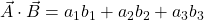# Vectors In Python/Numpy

How can we use numpy to solve generalized vector dot products such as the one below:

GivenandWhat is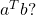Using python and the numpy library, we have two options for expressing this calculation, 1-D arrays, and matrices. But each has a caveat to consider.

In all code examples below assume we have imported the numpy library:


>>> import numpy as np

## Vector as 1-D Array

In python, using the numpy library, a vector can be represented as 1-D array or an Nx1 (or 1xN) matrix. For example:

>>> a = np.array([1,5,-3,2])       # create 1-D array, a simple list of numbers
>>> a
array([ 1,  5, -3,  2])
>>> a.shape
(4,)                               # shape is shown to be a 1-D array


If we take a transpose of the 1-D array, numpy will return the same dimension. So a transpose function has no effect on a numpy 1-D array.

>>> a
array([ 1,  5, -3,  2])
>>> a.shape               # shape of a is 4
(4,)
>>> at = a.transpose()

>>> at.shape
(4,)
>>> at
array([ 1,  5, -3,  2])  # shape of a-transpose is also 4

>>> at.shape
(4,)

So if we define a vector ‘a’ and a vector ‘b’ and try to find the dot product of the transpose of ‘a’ to ‘b’, the transpose will have no effect, but numpy will dot product the two single dimension vectors with this result:

>>> a
array([ 1,  5, -3,  2])
>>> b
array([8, 2, 4, 7])

>>> c = np.dot(a,b)   # take the dot product of 1-D vectors a and b
>>> c
20                     # the result is a scalar of value 20


Note the result is the expected value of 20, and it is a scalar as expected. So when using numpy 1-D arrays for dot products, the user has to be aware that transpose functions are meaningless but also will not affect the dot product result.

## Vector as a row/column of a 2-D Matrix

If we create the vector a as a numpy 2-D matrix by using the double brackets (single row, multi-column), the resulting matrix is shown below.

>>> a = np.array([ [1,5,-3,2]  ])   # single row, multi-column array with dimensions 1x4
>>> a
array([[ 1,  5, -3,  2]])
>>> a.shape
(1, 4)                              # shape is shown to be a NxM array with N=1, M=4


If we then take the transpose of a, we get:

>>> at = a.transpose()
>>> at                   # the transpose of a is now a 4x1 (4 row, 1 col) matrix
array([[ 1],
[ 5],
[-3],
[ 2]])
>>> at.shape
(4, 1)                   # shape is shown to be a NxM array with N=4, M=1



So back to our generalized problem (defined above), what is, using numpy matrices:

a = np.array([ [1,5,-3,2]  ]).transpose()  # implement a as given above
at = at.transpose()                        # get a transpose
b = np.array([ [8,2,4,7]  ]).transpose()   # implement b as given above
c = np.dot(at,b)                           # get the aT dot b
>>> c                                      # print the contents of c
array([])

We see that we can use the transpose as expected, and get the expected result of 20, but the result is expressed as a 1×1 matrix rather than the expected scaler.

Also, note, dot products of matrices are only defined as the product of matrices with orthogonal dimensions of (1xN dot Nx1), or (Nx1 dot 1xN). If you attempt to take the dot product of a Nx1 and Nx1, for example, you will get an error:

>>> c=np.dot(a,b)
Traceback (most recent call last):
File "<stdin>", line 1, in <module>
File "<__array_function__ internals>", line 6, in dot
ValueError: shapes (1,4) and (1,4) not aligned: 4 (dim 1) != 1 (dim 0)


# Conclusion

If numpy 1-D arrays are used for dot product, the user has to understand that transpose functions have no meaning. On the other hand, if numpy matrices are used, the transpose function has the expected meaning but the user has to remember to translate the 1×1 matrix result to a scaler result.